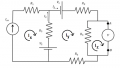# Kirchhoff Voltage law

#### gbox

Joined Dec 29, 2015
42How do I calculate the left loop?

From the one hand I have the current supplier but on the other hand a voltage supplier.

Should it be -R_1*I_so-R_3*I_so+R_3*I_2-V_2=0?

#### WBahn

Joined Mar 31, 2012
28,171
No.

You are doing a couple of things wrong. First, your loop equation isn't for a complete loop as it doesn't include the voltage across the current supply.

Second, you are mixing mesh currents and branch currents. You have both I_so and I_2 flowing in R3.

When doing mesh analysis, do mesh analysis. Forget branch currents entirely when setting up your equations - and label your mesh currents.

Note that you have THREE meshes:Set up your equations using only Ix, Iy, and Iz. Note, however, that the equations for Ix and Iz are pretty trivial. You can also simplify the equation for Iy by swapping the positions of the voltage source and R4, but even if you don't it is still pretty straightforward.

•gbox

#### gbox

Joined Dec 29, 2015
42
So I for I_x I have -I_so*R_1+I_2*R_3-V_2=0?

#### WBahn

Joined Mar 31, 2012
28,171
What did I say about branch currents? If you are doing mesh analysis, then do mesh analysis. Mesh analysis involves mesh currents, not branch currents. There should not be any I1 or I2 variables anywhere in your mesh equations because these are branch currents.

#### shteii01

Joined Feb 19, 2010
4,644
•gbox